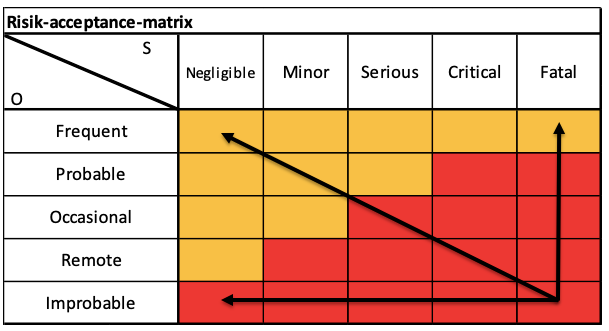top of page
Search

# How To Minimize Risks "as far as possible" in MedTech

Updated: Sep 5

The MDR requires in Annex I that medical device manufacturers must minimize risks "as far as possible".

But what exactly does that mean?

From how many measures is "as far as possible" achieved?

Where is the limit of "possible"?

Is it even economically possible to reduce risks "as far as possible"?

Does "as far as possible" mean that actually EVERYTHING possible must be done to minimize the occurrence and severity of damage?• Where is the Limit of Minimizing Risks "as far as possible"? How to solve the problem?

• Definition of to Minimize Risks "as far as possible"

## Where is the Limit of Minimizing Risks "as far as possible"? How to solve the problem?

In this context, the MDR stipulates that the "generally recognized state of the art" should be taken into account and that the "benefit-risk ratio" should not be negatively influenced. (Annex I, Chapter I, paragraphs 1 and 2)

It can therefore be assumed that risks are minimized "as far as possible" if measures are taken which correspond to the "generally acknowledged state of the art".

But what is meant by the "generally acknowledged state of the art"?## Definition of to Minimize Risks "as far as possible"

Unfortunately, there is currently no binding definition of the "generally acknowledged state of the art".

EN ISO 14971:2019 defines at least the "state of the art" as "generally accepted good practice in technology and medicine".

However, this shows that the "generally acknowledged state of the art" does not necessarily imply the most technically advanced solution. Applicable current norms, standards, statutory limits, industry standards, etc. constitute the "generally acknowledged state of the art".

## Conclusion

It can therefore be assumed that risks have been minimized "as far as possible" if mitigation measures have been taken that are based on the existing applicable norms, standards, etc.

It is not required that experimental or the most advanced technology is used to minimize risks "as far as possible".Author: Bernhard Lindner, MSc